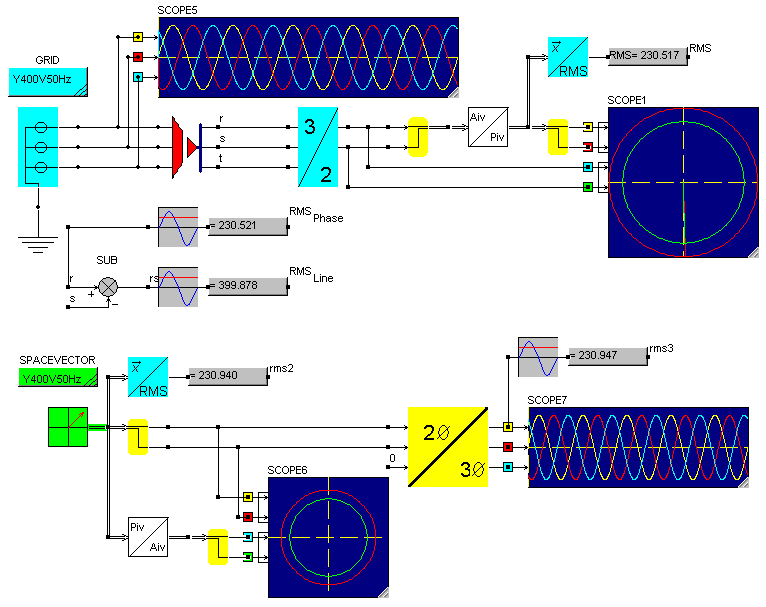• Introduction
• What is in this manual
• What is Caspoc
• User interface
• Introduction
• Starting
• Simulation
• Editing
• Viewing and printing
• Getting Started
• Basic editing
• Simulation in the time domain
• Basic User Interface Topics
• Editing
• Simulation
• Viewing
• Library
• Reports
• Project management
• Circuit and Block Diagram Components
• Introduction
• Cscript and user defined functions
• Component parameters
• Modeling Topics
• Introduction
• Power Electronics
• Semiconductors
• Electrical Machines
• Electrical drives
• Power Systems
• Mechanical Systems
• Thermal Systems
• Magnetic Circuits
• Green Energy
• Coupling to FEM
• Experimenter
• Analog hardware description language
• Embedded C code Export
• Coupling to Spice
• Small Signal Analysis
• Matlab coupling
• Tips and tricks
• Appendices

## Space vector compared to 3 phase.

The space vector models in the Advanced Electrical Drives library are power invariant models. Or better to say, the convention in the books is to use a power invariant transformation. This means that each component and connection is build on the basis of a vector, or better defined a space vector.
The space vector can be defined in the stator reference frame, rotor reference frame or any other reference frame. In many models the stator reference frame is used for the stator side of the model and the rotor reference frame is used for the rotor side of the model.

The simulation below compares the space vector with a three phase circuit, modeling a Y400V50Hz grid.The vector created directly from the voltage measurement and 3/2 transformation is in the Amplitude Invariant format. It is transformed into the Power Invariant format using the component AmplitudeInvariant2PowerInvariant from the Interface/General section.